Documentation

# unwrap

Shift phase angles

## Syntax

``Q = unwrap(P)``
``Q = unwrap(P,tol)``
``Q = unwrap(P,[],dim)``
``Q = unwrap(P,tol,dim)``

## Description

example

````Q = unwrap(P)` unwraps the radian phase angles in a vector `P`. Whenever the jump between consecutive angles is greater than or equal to π radians, `unwrap` shifts the angles by adding multiples of ±2π until the jump is less than π. If `P` is a matrix, `unwrap` operates columnwise. If `P` is a multidimensional array, `unwrap` operates on the first dimension whose size is larger than 1.```

example

````Q = unwrap(P,tol)` compares the jump between elements of `P` to the jump threshold `tol` instead of the default value π radians. If you specify a jump threshold less than π, unwrap uses the default jump threshold π.```

example

````Q = unwrap(P,[],dim)` unwraps along the dimension `dim`.```
````Q = unwrap(P,tol,dim)` unwraps along the dimension `dim` using the jump threshold `tol`.```

## Examples

collapse all

Define the $x$- and $y$-coordinates of a spiral with phase angle from 0 to $6\pi$. Plot the spiral.

```t = linspace(0,6*pi,201); x = t/pi.*cos(t); y = t/pi.*sin(t); plot(x,y)```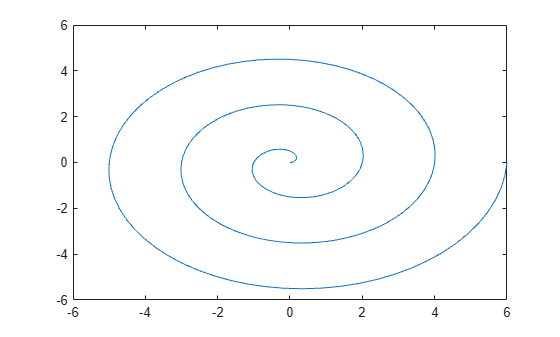Find the phase angle of the spiral from the $x$- and $y$-coordinates using the `atan2` function. The `atan2` function returns the angle values within the closed interval from $-\pi$ to $\pi$.

```P = atan2(y,x); plot(t,P)```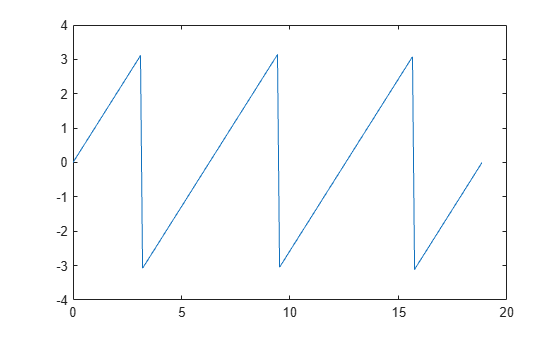Note that this plot has discontinuities. Use `unwrap` to eliminate the discontinuities. `unwrap` adds multiples of $±2\pi$ when the phase difference between consecutive elements of `P` are greater than or equal to the jump threshold $\pi$ radians. The shifted phase angle `Q` lies in the interval from 0 to $6\pi$.

```Q = unwrap(P); plot(t,Q)```Shift the phase angle of a frequency response. The phase curve has two jumps. The first jump is `3.4250` radians between `W = 3` and `W = 3.4`, and the second jump is `6.3420` radians between `W = 5` and `W = 5.4`. Plot the phase curve.

```clear; close all; W = [0:0.4:3, 3.4:0.4:5, 5.4:0.4:7]; P = [-1.5723 -1.5747 -1.5790 -1.5852 -1.5922 -1.6044 -1.6269 -1.6998 1.7252 1.5989 1.5916 1.5708 1.5582 -4.7838 -4.8143 -4.8456 -4.8764 -4.9002]; plot(W,P,'bo-')```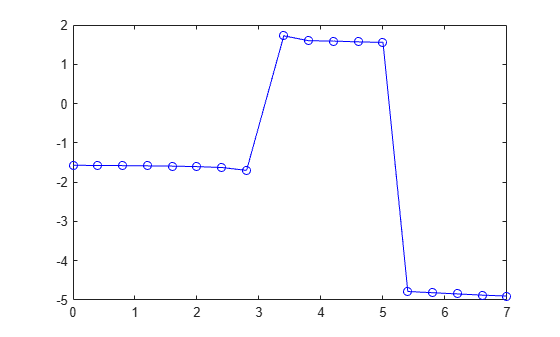Use `unwrap` to shift the phase angle using the default jump threshold $\pi$ radians. Plot the shifted phase curve. Both jumps are shifted since they are greater than the jump threshold $\pi$ radians.

`plot(W,unwrap(P),'ro-')`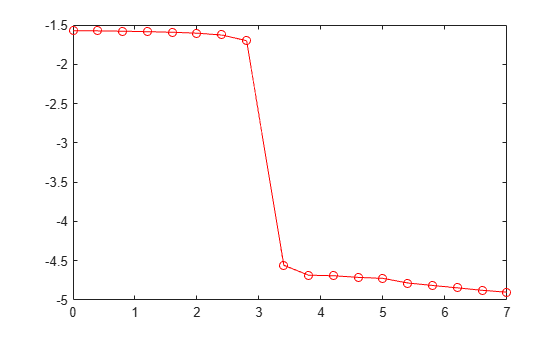Now shift the phase angle using a jump threshold of `5` radians. Plot the shifted phase curve. The first jump is not shifted since it is less than the jump threshold `5` radians.

`plot(W,unwrap(P,5),'ro-')`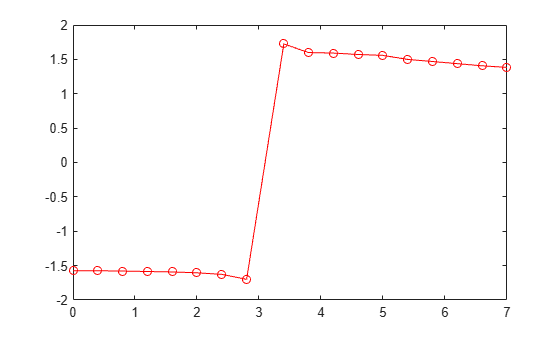Define a two-column matrix `P` that contains phase angles.

`P = [0 7.07; 0.19 0.98; 6.67 1.18; 0.59 1.37; 0.78 1.56]`
```P = 5×2 0 7.0700 0.1900 0.9800 6.6700 1.1800 0.5900 1.3700 0.7800 1.5600 ```

The phase angles `P(1,2) = 7.07` and `P(3,1) = 6.67` have phase differences that are larger than $\pi$ compared to the rest of the data.

Unwrap the phase angles by first comparing the elements columnwise. Specify the `dim` argument as 1. Use the default jump threshold $\pi$ by specifying the second argument as `[]`.

```dim = 1; P1 = unwrap(P,[],dim)```
```P1 = 5×2 0 7.0700 0.1900 7.2632 0.3868 7.4632 0.5900 7.6532 0.7800 7.8432 ```

To shift phase angles by rows instead of by columns, specify `dim` as 2 instead of 1.

```dim = 2; P2 = unwrap(P1,[],dim)```
```P2 = 5×2 0 0.7868 0.1900 0.9800 0.3868 1.1800 0.5900 1.3700 0.7800 1.5600 ```

## Input Arguments

collapse all

Input array, specified as a vector, matrix, or multidimensional array.

Data Types: `single` | `double`

Jump threshold to apply phase shift, specified as a scalar. A jump threshold less than π has the same effect as the default threshold π.

Data Types: `single` | `double`

Dimension to operate along, specified as a positive integer scalar. If no value is specified, then the default is the first array dimension whose size does not equal 1.

• `unwrap(P,[],1)` operates along the columns of `P` and returns the shifted phase angle of each column.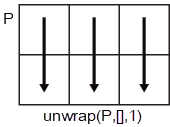• `unwrap(P,[],2)` operates along the rows of `P` and returns the shifted phase angle of each row.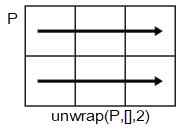If `dim` is greater than `ndims(P)`, then `unwrap(P,[],dim)` returns `P`.

Data Types: `single` | `double` | `int8` | `int16` | `int32` | `int64` | `uint8` | `uint16` | `uint32` | `uint64`

## Output Arguments

collapse all

Shifted phase angle, returned as a vector, matrix, or multidimensional array. The size of the output `Q` is always the same as the size of the input `P`.

Data Types: `single` | `double`